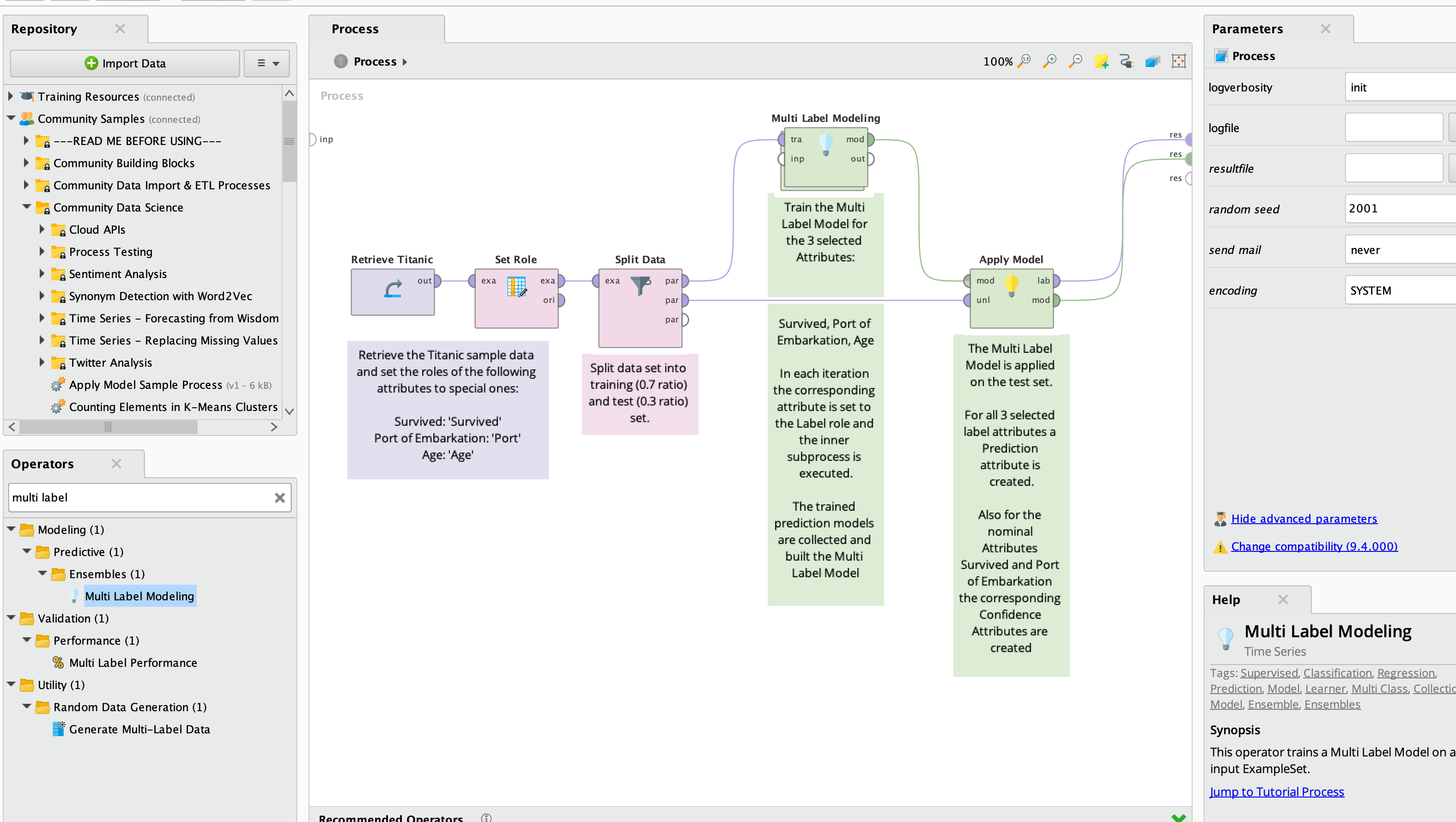# Vector Linear Regression - Multi Label

Member Posts: 35Contributor II
edited December 2019 in Help
Hello,
I'm trying to built a vector linear regression model with multiple label attributes, but I'm finding difficulties with the special role setup.

I read the vector linear regression description and from the below I understand that all labels must be named in its special role as something like "label_1", "label_2" and so on.

****The attributes forming the vector should be marked as special, the special role names of all label attributes should start with 'label'.*****

I'm sure that this must be easy, but whatever I've tried doesn't work.

Enclosed the example process and the data

Can you please shed some light on this?

Thanks

Tagged:

• 12Administrator, Moderator, Employee, RapidMiner Certified Analyst, Community Manager, Member, University Professor, PM Moderator Posts: 2,717Community Manager
hi @Oprick no you need to only label one target at a time.

```<?xml version="1.0" encoding="UTF-8"?><process version="9.5.001">
<context>
<input/>
<output/>
<macros/>
</context>
<operator activated="true" class="process" compatibility="9.5.001" expanded="true" name="Process">
<parameter key="logverbosity" value="init"/>
<parameter key="random_seed" value="2001"/>
<parameter key="send_mail" value="never"/>
<parameter key="process_duration_for_mail" value="30"/>
<parameter key="encoding" value="SYSTEM"/>
<process expanded="true">
<parameter key="excel_file" value="example.xlsx"/>
<parameter key="sheet_selection" value="sheet number"/>
<parameter key="sheet_number" value="1"/>
<parameter key="imported_cell_range" value="A1"/>
<parameter key="encoding" value="SYSTEM"/>
<parameter key="first_row_as_names" value="true"/>
<list key="annotations"/>
<parameter key="date_format" value=""/>
<parameter key="time_zone" value="SYSTEM"/>
<parameter key="locale" value="English (United States)"/>
<list key="data_set_meta_data_information">
<parameter key="0" value="A.true.integer.attribute"/>
<parameter key="1" value="B.true.integer.attribute"/>
<parameter key="2" value="C.true.integer.attribute"/>
<parameter key="3" value="D.true.integer.attribute"/>
<parameter key="4" value="E.true.integer.attribute"/>
<parameter key="5" value="year.true.integer.attribute"/>
<parameter key="6" value="week.true.integer.attribute"/>
<parameter key="7" value="air.true.real.attribute"/>
<parameter key="8" value="mmp.true.real.attribute"/>
<parameter key="9" value="total.true.integer.attribute"/>
<parameter key="10" value="total+1.true.integer.attribute"/>
<parameter key="11" value="label_A+1.true.integer.attribute"/>
<parameter key="12" value="label_B+1.true.integer.attribute"/>
<parameter key="13" value="label_C+1.true.integer.attribute"/>
<parameter key="14" value="label_D+1.true.integer.attribute"/>
<parameter key="15" value="label_E+1.true.integer.attribute"/>
<parameter key="16" value="total-1.true.integer.attribute"/>
<parameter key="17" value="total-2.true.integer.attribute"/>
<parameter key="18" value="total-3.true.integer.attribute"/>
<parameter key="19" value="A-1.true.integer.attribute"/>
<parameter key="20" value="A-2.true.integer.attribute"/>
<parameter key="21" value="A-3.true.integer.attribute"/>
<parameter key="22" value="B-1.true.integer.attribute"/>
<parameter key="23" value="B-2.true.integer.attribute"/>
<parameter key="24" value="B-3.true.integer.attribute"/>
<parameter key="25" value="C-1.true.integer.attribute"/>
<parameter key="26" value="C-2.true.integer.attribute"/>
<parameter key="27" value="C-3.true.integer.attribute"/>
<parameter key="28" value="D-1.true.integer.attribute"/>
<parameter key="29" value="D-2.true.integer.attribute"/>
<parameter key="30" value="D-3.true.integer.attribute"/>
<parameter key="31" value="E-1.true.integer.attribute"/>
<parameter key="32" value="E-2.true.integer.attribute"/>
<parameter key="33" value="E-3.true.integer.attribute"/>
<parameter key="34" value="id.true.integer.attribute"/>
</list>
<parameter key="datamanagement" value="double_array"/>
<parameter key="data_management" value="auto"/>
</operator>
<operator activated="true" class="set_role" compatibility="9.5.001" expanded="true" height="82" name="Set Role" width="90" x="179" y="34">
<parameter key="attribute_name" value="label_A+1"/>
<parameter key="target_role" value="label"/>
</operator>
<operator activated="true" class="vector_linear_regression" compatibility="9.5.001" expanded="true" height="82" name="Vector Linear Regression" width="90" x="313" y="34">
<parameter key="use_bias" value="true"/>
<parameter key="ridge" value="1.0E-8"/>
</operator>
<operator activated="true" class="apply_model" compatibility="9.5.001" expanded="true" height="82" name="Apply Model" width="90" x="447" y="34">
<list key="application_parameters"/>
<parameter key="create_view" value="false"/>
</operator>
<connect from_op="Read Excel" from_port="output" to_op="Set Role" to_port="example set input"/>
<connect from_op="Set Role" from_port="example set output" to_op="Vector Linear Regression" to_port="training set"/>
<connect from_op="Vector Linear Regression" from_port="model" to_op="Apply Model" to_port="model"/>
<connect from_op="Vector Linear Regression" from_port="exampleSet" to_op="Apply Model" to_port="unlabelled data"/>
<connect from_op="Apply Model" from_port="labelled data" to_port="result 2"/>
<connect from_op="Apply Model" from_port="model" to_port="result 1"/>
<portSpacing port="source_input 1" spacing="0"/>
<portSpacing port="sink_result 1" spacing="0"/>
<portSpacing port="sink_result 2" spacing="0"/>
<portSpacing port="sink_result 3" spacing="0"/>
</process>
</operator>
</process>
```

Scott
• Member Posts: 35Contributor II
Hi @sgenzer,
when in VLR operator's description says (see below) I have to confess that the plural on word label lead me to believe that this operator was capable of dealing with multi-label problems.

"It regresses all regular attributes upon a vector of labels. The attributes forming the vector should be marked as special, the special role names of all label attributes should start with 'label'."

Thanks,
Pedro

• 12Administrator, Moderator, Employee, RapidMiner Certified Analyst, Community Manager, Member, University Professor, PM Moderator Posts: 2,717Community Manager
edited December 2019
hi @Oprick in all honesty I have never used that operator in 6+ years of using the software. Never even seen it before. Looking at the tutorial it seems to function like the other learners but I do see what you're saying. I just opened up the source code on GitHub and it looks like our good friend @TobiasMalbrecht was the author back in the day. Maybe he has some insight...If you want multi-label modeling, I'd just use the multi-labeling modeling operator in the Ensembles folder. Open the tutorial and you will see how it works on Titanic.Scott

• Member Posts: 35Contributor II
Hi @sgenzer, thanks for you help and pulling the Tobias into the thread.
I'm aware of multi-label indeed, but I have to say that I'm particular curious of this supposed capabilities of VLR.

• 12Administrator, Moderator, Employee, RapidMiner Certified Analyst, Community Manager, Member, University Professor, PM Moderator Posts: 2,717Community Manager
fair enough @Oprick. You will have to wait for the maestro @TobiasMalbrecht to get back from vacation• Member Posts: 35Contributor II